## Exercise 11.1 Page No: 171

1.A square and a rectangular field with measurements as given in the figure have the same perimeter. Which field has a larger area?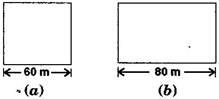Solution:

Side of a square = 60 m (Given)

And the length of rectangular field, l = 80 m (Given)

According to question,

Perimeter of rectangular field = Perimeter of square field

2(l+b) = 4×Side (using formulas)

2(80+b) = 4×60

160+2b = 240

b = 40

Breadth of the rectangle is 40 m.

Now, Area of Square field

= (side)2

= (60)2 = 3600 m2

And Area of Rectangular field

= 3200 m2

Hence, area of square field is larger.

2. Mrs.Kaushik has a square plot with the measurement as shown in the figure. She wants to construct a house in the middle of the plot. A garden is developed around the house. Find the total cost of developing a garden around the house at the rate of Rs. 55 per m2.Solution:

Side of a square plot = 25 m

Formula: Area of square plot =  square of a side = (side)2

= (25)2 = 625

Therefore the area of a square plot is 625 m2

Length of the house = 20 m and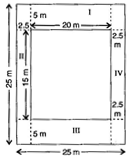Breadth of the house = 15 m

Area of the house = length×breadth

= 20×15 = 300 m2

Area of garden = Area of square plot – Area of house

= 625–300 = 325 m2

∵ Cost of developing the garden per sq. m is Rs. 55

Cost of developing the garden 325 sq. m = Rs. 55×325

= Rs. 17,875

Hence total cost of developing a garden around is Rs. 17,875.

3. The shape of a garden is rectangular in the middle and semi-circular at the ends as shown in the diagram. Find the area and the perimeter of this garden [Length of rectangle is 20 – (3.5 + 3.5 meters]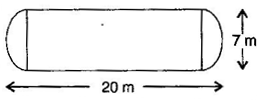Solution::

Given: Total length = 20 m

Diameter of semi circle = 7 m

Radius of semi circle = 7/2 = 3.5 m

Length of rectangular field

= 20-(3.5+3.5) = 20-7 = 13 m

Breadth of the rectangular field = 7 m

Area of rectangular field = l×b

= 13×7= 91m2

Area of two semi circles = 2×(1/2)×π×r2

= 2×(1/2)×22/7×3.5×3.5

= 38.5 m2

Area of garden = 91+38.5 = 129.5 m2

Now Perimeter of two semi circles =  2πr = 2×(22/7)×3.5  = 22 m

And Perimeter of garden = 22+13+13

4. A flooring tile has the shape of a parallelogram whose base is 24 cm and the corresponding height is 10 cm. How many such tiles are required to cover a floor of area 1080 m2? [If required you can split the tiles in whatever way you want to fill up the corners]

Solution:

Given: Base of flooring tile = 24 cm = 0.24 m

Corresponding height of a flooring tile= 10 cm = 0.10 m

Now Area of flooring tile= Base×Altitude

= 0.24×0.10

= 0.024

Area of flooring tile is 0.024m2

Number of tiles required to cover the floor= Area of floor/Area of one tile =  1080/0.024

= 45000 tiles

Hence 45000 tiles are required to cover the floor.

5. An ant is moving around a few food pieces of different shapes scattered on the floor. For which food-piece would the ant have to take a longer round? Remember, circumference of a circle can be obtained by using the expression C = 2πr ,where  r  is the radius of the circle.Solution:

(a) Radius =  Diameter/2 = 2.8/2 cm = 1.4 cm

Circumference of semi-circle =  πr

=  (22/7)×1.4 = 4.4

Circumference of semi-circle is 4.4 cm

Total distance covered by the ant= Circumference of semi -circle+Diameter

= 4.4+2.8 = 7.2 cm

(b) Diameter of semi-circle = 2.8 cm

Radius =  Diameter/2 = 2.8/2  = 1.4 cm

Circumference of semi-circle = r

= (22/7)×1.4 = 4.4 cm

Total distance covered by the ant= 1.5+2.8+1.5+4.4 = 10.2 cm

(c) Diameter of semi-circle = 2.8 cm

= 1.4 cm

Circumference of semi-circle =  π r

=  (22/7)×1.4

= 4.4 cm

Total distance covered by the ant = 2+2+4.4 = 8.4 cm

After analyzing results of three figures, we concluded that for figure (b) food piece, the ant would take a longer round.

## Exercise 11.2 Page No: 177

1.The shape of the top surface of a table is a trapezium. Find its area if its parallel sides are 1 m and 1.2 m and perpendicular distance between them is 0.8 m.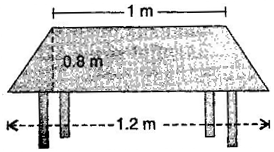Solution: One parallel side of the trapezium (a) = 1 m

And second side (b) = 1.2 m and

height  (h) = 0.8 m

Area of top surface of the table=  (½)×(a+b)h

= (½)×(1+1.2)0.8

=  (½)×2.2×0.8 = 0.88

Area of top surface of the table is 0.88 m2 .

2. The area of a trapezium is 34  cm2and the length of one of the parallel sides is 10 cm and its height is 4 cm Find the length of the other parallel side.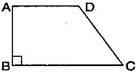Solution: Let the length of the other parallel side be b.

Length of one parallel side, a = 10 cm

height, (h) = 4 cm and

Area of a trapezium is 34 cm2

Formula for, Area of trapezium = (1/2)×(a+b)h

34 = ½(10+b)×4

34 = 2×(10+b)

After simplifying, b = 7

Hence another required parallel side is 7 cm.

3. Length of the fence of a trapezium shaped field ABCD is 120 m. If BC = 48 m, CD = 17 m and AD = 40 m, find the area of this field. Side AB is perpendicular to the parallel sides AD and BC.

Solution: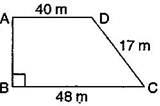Given: BC = 48 m, CD = 17 m,

AD = 40 m and perimeter = 120 m

∵ Perimeter of trapezium ABCD

= AB+BC+CD+DA

120 = AB+48+17+40

120 = AB = 105

AB = 120–105 = 15 m

Now, Area of the field=  (½)×(BC+AD)×AB

= (½)×(48 +40)×15

= (½)×88×15

= 660

Hence, area of the field ABCD is 660m.

4. The diagonal of a quadrilateral shaped field is 24 m and the perpendiculars dropped on it from the remaining opposite vertices are 8 m and 13 m. Find the area of the field.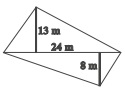Solution:Consider, h1 = 13 m, h2 = 8 m and AC = 24 m

Area of quadrilateral ABCD = Area of triangle ABC+Area of triangle ADC

= ½( bh1)+ ½(bh2)

= ½ ×b(h1+h2)= (½)×24×(13+8)

= (½)×24×21 = 252

Hence, required area of the field is 252 m2

5. The diagonals of a rhombus are 7.5 cm and 12 cm. Find its area.

Solution:

Given:  d1 = 7.5 cm and d2 = 12 cm

We know that,Area of rhombus =  (½ )×d1×d2

= (½)×7.5×12 = 45

Therefore, area of rhombus is 45 cm2 .

6. Find the area of a rhombus whose side is 5 cm and whose altitude is 4.8 cm. If one of the diagonals is 8 cm long, find the length of the other diagonal.

Solution: Since a rhombus is also a kind of a parallelogram.

Formula for Area of rhombus = Base×Altitude

Putting values, we have

Area of rhombus = 6×4 = 24

Area of rhombus is 24 cm2

Also, Formula for Area of rhombus = (½)×d1d2

After substituting the values, we get

24 = (½)×8×d2

d2 = 6

Hence, the length of the other diagonal is 6 cm.

7. The floor of a building consists of 3000 tiles which are rhombus shaped and each of its diagonals are 45 cm and 30 cm in length. Find the total cost of polishing the floor, if the cost per m2is Rs. 4.

Solution:

Length of one diagonal,  d1 = 45 cm and  d2= 30 cm

∵ Area of one tile =  (½)d1d2

=  (½)×45×30 = 675

Area of one tile is 675 cm2

Area of 3000 tiles is

= 675×3000 = 2025000 cm2

= 2025000/10000

= 202.50 m[∵ 1m2 = 10000 cm2]

∵ Cost of polishing the floor per sq. meter = 4

Cost of polishing the floor per 202.50 sq. meter = 4×202.50 = 810

Hence the total cost of polishing the floor is Rs. 810.

8. Mohan wants to buy a trapezium shaped field. Its side along the river is parallel to and twice the side along the road. If the area of this field is 10500 m2 and the perpendicular distance between the two parallel sides is 100 m, find the length of the side along the river.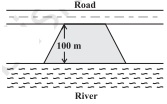Solution:

Perpendicular distance  (h) = 100 m (Given)

Area of the trapezium shaped field = 10500 m2 (Given)

Let side along the road be ‘x’ m and side along the river =  2x m

Area of the trapezium field =  (½)×(a+b)×h

10500 =  (½)×(x+2x)×100

10500 = 3x×50

After simplifying, we have x = 70, which means side along the river is 70 m

Hence, the side along the river =  2x = 2( 70) = 140 m.

9. Top surface of a raised platform is in the shape of a regular octagon as shown in the figure. Find the area of the octagonal surface.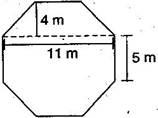Solution:

Octagon having eight equal sides, each 5 m. (given)

Divide the octagon as show in the below figure, 2 trapeziums whose parallel and perpendicular sides are 11 m and 4 m respectively and 3rd one is rectangle having length and breadth 11 m and 5 m respectively.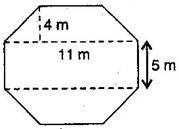Now, Area of two trapeziums = 2 [(½)×(a+b)×h]

= 2×(½)×(11+5 )×4

= 4×16 = 64

Area of two trapeziums is 64 m2

Also, Area of rectangle = length×breadth

= 11×5 = 55

Area of rectangle is 55 m2

Total area of octagon = 64+55

= 119 m2

10. There is a pentagonal shaped park as shown in the figure.

For finding its area Jyoti and Kavita divided it in two different ways.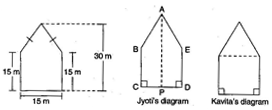Find the area of this park using both ways. Can you suggest some other way of finding its area?

Solution:

First way:  By Jyoti’s diagram,

Area of pentagon = Area of trapezium ABCP + Area of trapezium AEDP

=  (½)(AP+BC)×CP+(1/2)×(ED+AP)×DP

=  (½)(30+15)×CP+(1/2)×(15+30)×DP

=  (½)×(30+15)×(CP+DP)

=  (½)×45×CD

= (1/2)×45×15

=337.5 m2

Area of pentagon is 337.5 m2

Second way: By Kavita’s diagramHere, a perpendicular AM drawn to BE.

AM = 30–15 = 15 m

Area of pentagon = Area of  triangle ABE+Area of square BCDE (from above figure)

= (½)×15×15+(15×15)

= 112.5+225.0

= 337.5

Hence, total area of pentagon shaped park = 337.5 m2

11. Diagram of the adjacent picture frame has outer dimensions = 24 cm×28 cm and inner dimensions 16 cm×20 cm. Find the area of each section of the frame, if the width of each section is same.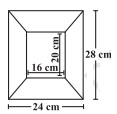Solution:

Divide given figure into 4 parts, as shown below: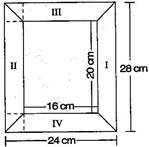Here two of given figures (I) and (II) are similar in dimensions.

And also figures (III) and (IV) are similar in dimensions.

Area of figure (I) = Area of trapezium

=  (½)×(a+b)×h

= (½)×(28+20)×4

=  (½)×48×4 = 96

Area of figure (I) = 96 cm2

Also,Area of figure (II) = 96 cm2

Now, Area of figure (III) = Area of trapezium

= (½)×(a+b)×h

= (½)×(24+16)4

= (½)×40×4 = 80

Area of figure (III) is 80 cm2

Also, Area of figure (IV) = 80 cm2

## Exercise 11.3 Page No: 186

1. There are two cuboidal boxes as shown in the adjoining figure. Which box requires the lesser amount of material to make?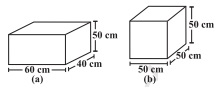Solution:

(a) Given: Length of cuboidal box  (l) = 60 cm

Breadth of cuboidal box  (b)  = 40 cm

Height of cuboidal box  (h)  = 50 cm

Total surface area of cuboidal box =  2×(lb+bh+hl)

= 2×(60×40+40×50+50×60)

= 2×(2400+2000+3000)

= 14800 cm2

(b)  Length of cubical box (l) = 50 cm

Breadth of cubicalbox (b) = 50 cm

Height of cubicalbox (h) = 50 cm

Total surface area of cubical box = 6(side)2

= 6(50×50)

= 6×2500

= 15000

Surface area of the cubical box is 15000 cm2

From the result of (a) and (b), cuboidal box requires the lesser amount of material to make.

2. A suitcase with measures 80 cm x 48 cm x 24 cm is to be covered with a tarpaulin cloth. How many meters of tarpaulin of width 96 cm is required to cover 100 such suitcases?

Solution:

Length of suitcase box, l = 80 cm,

Breadth of suitcase box, b= 48 cm

And Height of cuboidal box , h = 24 cm

Total surface area of suitcase box = 2(lb+bh+hl)

= 2(80×48+48×24+24×80)

= 2 (3840+1152+1920)

= 2×6912

= 13824

Total surface area of suitcase box is 13824 cm2

Area of Tarpaulin cloth = Surface area of suitcase

l×b = 13824

l ×96 = 13824

l = 144

Required tarpaulin for 100 suitcases = 144×100 = 14400 cm = 144 m

Hence tarpaulin cloth required to cover 100 suitcases is 144 m.

3. Find the side of a cube whose surface area is 600cm^2 .

Solution:

Surface area of cube = 600 cm2 (Given)

Formula for surface area of a cube = 6(side)2

Substituting the values, we get

6(side)2 = 600

(side)2 = 100

Or side = ±10

Since side cannot be negative, the measure of each side of a cube is 10 cm

4. Rukshar painted the outside of the cabinet of measure 1 m ×2 m ×1.5 m. How much surface area did she cover if she painted all except the bottom of the cabinet?Solution:

Length of cabinet, l = 2 m, Breadth of cabinet, b = 1 m and Height of cabinet, h  = 1.5 m

Surface area of cabinet =  lb+2(bh+hl )

= 2×1+2(1×1.5+1.5×2)

= 2+2(1.5+3.0)

= 2+9.0

= 11

Required surface area of cabinet is 11m2.

5. Daniel is paining the walls and ceiling of a cuboidal hall with length, breadth and height of 15 m, 10 m and 7 m respectively. From each can of paint 100 m^2of area is painted. How many cans of paint will she need to paint the room?

Solution:

Length of wall, l  = 15 m, Breadth of wall, b = 10 m and Height of wall, h  = 7 m

Total Surface area of classroom = lb+2(bh+hl )

= 15×10+2(10×7+7×15)

= 150+2(70+105)

= 150+350

= 500

Now, Required number of cans =  Area of hall/Area of one can

= 500/100 = 5

Therefore, 5 cans are required to paint the room.

6. Describe how the two figures below are alike and how they are different. Which box has larger lateral surface areas?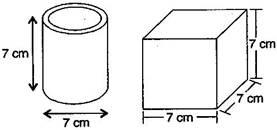Solution:

Diameter of cylinder = 7 cm (Given)

Radius of cylinder, r  =  7/2  cm

Height of cylinder, h = 7 cm

Lateral surface area of cylinder = 2πrh

=  2×(22/7)×(7/2)×7 = 154

So, Lateral surface area of cylinder is154 cm2

Now, lateral surface area of cube =  4 (side)2=4×72 = 4×49 = 196

Lateral surface area of cube is 196 cm2

Hence, the cube has larger lateral surface area.

7. A closed cylindrical tank of radius 7 m and height 3 m is made from a sheet of metal. How much sheet of metal is required?

Solution: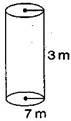Radius of cylindrical tank, r  = 7 m

Height of cylindrical tank , h = 3 m

Total surface area of cylindrical tank = 2πr(h+r)

=  2×(22/7)×7(3+7)

= 44×10 = 440

Therefore, 440  m2 metal sheet is required.

8. The lateral surface area of a hollow cylinder is 4224cm2. It is cut along its height and formed a rectangular sheet of width 33 cm. Find the perimeter of rectangular sheet?

Solution:

Lateral surface area of hollow cylinder = 4224  cm2

Width of rectangular sheet = 33 cm and say l be the length of the rectangular sheet

Lateral surface area of cylinder = Area of rectangular sheet

4224 =  b × l

4224 = 33 × l

l = 4224/33 = 128 cm

So the length of the rectangular sheet is 128 cm.

Also, Perimeter of rectangular sheet =  2(l+b)

= 2(128+33)

= 322 cm

The perimeter of rectangular sheet is 322 cm.

9. A road roller takes 750 complete revolutions to move once over to level a road. Find the area of the road if the diameter of a road roller is 84 cm and length 1 m.Solution:

Diameter of road roller, d = 84 cm

Radius of road roller, r = d/2 = 84/2 = 42 cm

Length of road roller, h = 1 m = 100 cm

Formula for Curved surface area of road roller = 2πrh

= 2×(22/7)×42×100 = 26400

Curved surface area of road roller is 26400 cm2

Again, Area covered by road roller in 750 revolutions = 26400×750cm2

= 1,98,00,000cm2

= 1980 m2  [∵ 1 m2= 10,000 cm2]

Hence the area of the road is 1980 m2.

10. A company packages its milk powder in cylindrical container whose base has a diameter of 14 cm and height 20 cm. Company places a label around the surface of the container (as shown in figure). If the label is placed 2 cm from top and bottom, what is the area of the label?Solution:

Diameter of cylindrical container , d = 14 cm

Radius of cylindrical container, r = d/2 = 14/2  = 7 cm

Height of cylindrical container = 20 cm

Height of the label, say h = 20–2–2 (from the figure)

= 16 cm

Curved surface area of label = 2πrh

=  2×(22/7)×7×16

= 704

Hence, the area of the label is 704 cm2.

## Exercise 11.4 Page No: 191

1.Given a cylindrical tank, in which situation will you find surface are and in which situation volume.

(a) To find how much it can hold.

(b) Number of cement bags required to plaster it.

(c) To find the number of smaller tanks that can be filled with water from it.

Solution: We find area when a region covered by a boundary, such as outer and inner surface area of a cylinder, a cone, a sphere and surface of wall or floor.

When the amount of space occupied by an object such as water, milk, coffee, tea, etc., then we have to find out volume of the object.

(a) Volume (b) Surface area (c) Volume

2. Diameter of cylinder A is 7 cm and the height is 14 cm. Diameter of cylinder B is 14 cm and height is 7 cm. Without doing any calculations can you suggest whose volume is greater? Verify it by finding the volume of both the cylinders. Check whether the cylinder with greater volume also has greater surface area.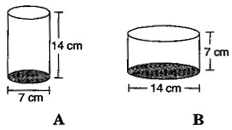Solution:

Yes, we can say that volume of cylinder B is greater, since radius of cylinder B is greater than that of cylinder A.

Find Volume for cylinders A and B

Diameter of cylinder A = 7 cm

Radius of cylinder A = 7/2  cm

And Height of cylinder A = 14 cm

Volume of cylinder A = πr2h

=  (22/7 )×(7/2)×(7/2)×14 = 539

Volume of cylinder A is 539  cm3

Now, Diameter of cylinder B = 14 cm

Radius of cylinder B =  14/2 = 7 cm

And Height of cylinder B = 7 cm

Volume of cylinder B = πr2h

= (22/7)×7×7×7 = 1078

Volume of cylinder B is 1078 cm3

Find surface area for cylinders A and B

Surface area of cylinder A =  2πr(r+h )

= 2 x 22/7 x 7/2 x (7/2 + 14) = 385

Surface area of cylinder A is385 cm2

Surface area of cylinder B =  2πr(r+h)

= 2×(22/7)×7(7+7) = 616

Surface area of cylinder B is 616 cm2

Yes, cylinder with greater volume also has greater surface area.

3. Find the height of a cuboid whose base area is 180 cm2 and volume is 900 cm3?

Solution:

Given, Base area of cuboid = 180 cm2 and Volume of cuboid = 900 cm3

We know that, Volume of cuboid = lbh

900 = 180×h (substituting the values)

h= 900/180 = 5

Hence the height of cuboid is 5 cm.

4. A cuboid is of dimensions 60 cm×54 cm×30 cm. How many small cubes with side 6 cm can be placed in the given cuboid?

Solution:

Given, Length of cuboid, l = 60 cm, Breadth of cuboid, b = 54 cm and

Height of cuboid, h = 30 cm

We know that, Volume of cuboid = lbh = 60 ×54×30  = 97200 cm3

And Volume of cube = (Side)3

= 6×6×6 = 216 cm3

Also, Number of small cubes =  volume of cuboid / volume of cube

= 97200/216

= 450

Hence , required cubes are 450.

5. Find the height of the cylinder whose volume if 1.54 m3 and diameter of the base is 140 cm.

Solution:

Given: Volume of cylinder = 1.54 m3and Diameter of cylinder = 140 cm

Radius  ( r )=  d/2 = 140/2  = 70 cm

Volume of cylinder = πr2h

1.54 = (22/7)×0.7×0.7×h

After simplifying, we get the value of h that is

h = (1.54×7)/(22×0.7×0.7)

h = 1

Hence, height of the cylinder is 1 m.

6. A milk tank is in the form of cylinder whose radius is 1.5 m and length is 7 m. Find the quantity of milk in liters that can be stored in the tank.Solution:

Given, Radius of cylindrical tank, r = 1.5 m and Height of cylindrical tank, h  = 7 m

Volume of cylindrical tank, V =  πr2h

= (22/7)×1.5×1.5 ×7

= 49.5  cm3

= 49.5×1000 liters = 49500 liters

[∵ 1 m3= 1000 liters]

Hence, required quantity of milk is 49500 liters.

7. If each edge of a cube is doubled,

(i) how many times will its surface area increase?

(ii) how many times will its volume increase?

Solution:

(i) Let the edge of cube be “ l” .

Formula for Surface area of the cube, A =  6 l2

When edge of cube is doubled, then

Surface area of the cube, say A’ = 6(2l)2 = 6×4l2 = 4(6 l2)

A’ = 4A

Hence, surface area will increase by four times.

(ii) Volume of cube, V = l3

When edge of cube is doubled, then

Volume of cube, say V’ = (2l)3 = 8( l3)

V’ = 8×V

Hence, volume will increase 8 times.

8. Water is pouring into a cuboidal reservoir at the rate of 60 liters per minute. If the volume of reservoir is 108 m^3, find the number of hours it will take to fill the reservoir.

Solution:

Given, volume of reservoir = 108 m3

Rate of pouring water into cuboidal reservoir = 60 liters/minute

=  60/1000  m3per minute

Since 1 liter = (1/1000 )m3

=  (60×60)/1000 m3 per hour

Therefore, (60×60)/1000 m3 water filled in reservoir will take = 1 hour

Therefore 1 m3 water filled in reservoir will take =  1000/(60×60)  hours

Therefore, 108 m3 water filled in reservoir will take = (108×1000)/(60×60)  hours = 30 hours

Answer: It will take 30 hours to fill the reservoir.

### NCERT Solutions for Class 8 Maths Chapter 11 Mensuration

In the previous grade, students learnt about area and perimeter of closed plane figures such as circles, squares, triangles, rectangles etc. In Class 8 CBSE Maths Chapter 11, students will learn about surface area and volume of solids such as cube, cuboid and cylinder, area of trapezium, area of quadrilateral, area of special quadrilaterals, area of a polygon, solid shapes, volume and capacity. Students can access this chapter’s NCERT Solutions for Class 8 while solving the exercise problems.

Try NCERT Solutions for Class 8 Maths Chapter 11 problems with answers to understand the concept, because Mensuration is a topic widely used in real-life applications. All the NCERT Solutions are designed by subject experts according to the latest CBSE Syllabus to help students fetch high marks.

The topics discussed in the chapter revolves around:

1. Area of:
(i) a trapezium = half of the sum of the lengths of parallel sides × perpendicular distance between
them.
(ii) a rhombus = half the product of its diagonals.
2. Surface area of a solid is the sum of the areas of its faces.
3. Surface area of
a cuboid = 2(lb + bh + hl)
a cube = 6l2
a cylinder = 2πr(r + h)
4. Amount of region occupied by a solid is called its volume.
5. Volume of
a cuboid = l × b × h
a cube = l3
a cylinder = πr2h
6. (i) 1 cm3= 1 mL
(ii) 1L = 1000 cm3
(iii) 1 m= 1000000 cm= 1000L

#### The main topics covered in this chapter include:

 Exercise Topic 11.1 Introduction 11.2 Let us Recall 11.3 Area of Trapezium 11.4 Area of a General Quadrilateral 11.5 Area of a Polygon 11.6 Solid Shapes 11.7 Surface Area of Cube, Cuboid and Cylinder 11.8 Volume of Cube, Cuboid and Cylinder 11.9 Volume and Capacity

Disclaimer:

Dropped Topics – 11.2 Let us Recall, 11.3 Area of Trapezium and 11.4 Area of a General Quadrilateral.